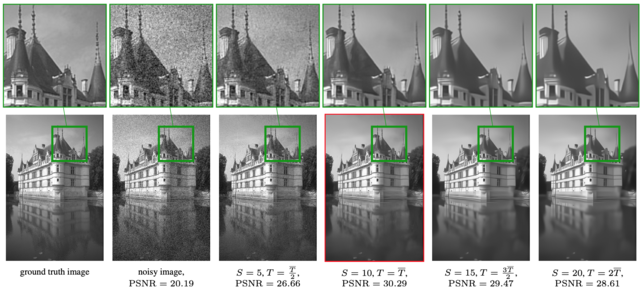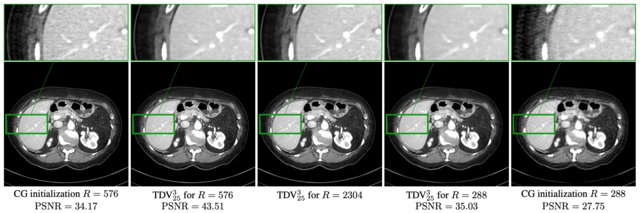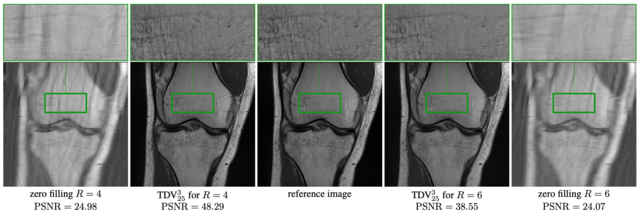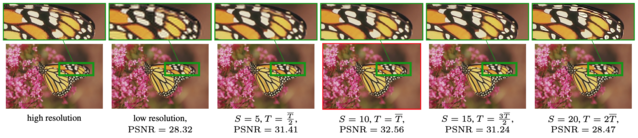# Popular Science

Do you think the above picture could depict paintings in a contemporary art exhibition? What are they allegorising? What was the painter’s intention? How is a contemporary art painting characterised anyway? The paintings have a ‘futuristic’ look, many geometric objects, homogeneous regions, greyscale colours. But does that make them pieces of art?

Or is it possible that those images were created by ‘artificial intelligence’? What criteria would you consider to judge?

In fact, the three images above are eigenfunctions resulting from calculations done in the context of an ‘AI’ framework. More specifically, in the 2020 CVPR paper on 'Total Deep Variation for Linear Inverse Problems' inverse imaging problems such as denoising are cast as variational problems with a learnable regulariser called Total Deep Variation (TDV). The learning problem is written as a discretely sampled optimal control problem for which the adjoint state equations and an optimality condition are derived.

The schematic on the left breaks down the components of the TDV regulariser from a high-level view (top) down to detailing the low-level operations. On the highest level, wTN(Kx) assigns to each pixel an energy value incorporating the local neighbourhood, where w is a learnt weight vector and K is a learnt convolution kernel. The function N (yellow) is composed of three macro-blocks (red), each representing a CNN with a U-Net-type architecture. The macro-blocks consist of five micro-blocks (blue) with a residual structure on three scales.

TDV is defined as the total sum of the pixel-wise regularisation depicted above. In order to analyse the local behaviour of TDV for a denoising problem with fixed noise level, a saddle point of the Lagrangian of TDV is computed and turns out to be a non-linear eigenpair of the gradient of TDV.

In the figure above you can recognise the three ‘paintings’ among others. They are in fact eigenfunctions (second row) of input images to the denoising framework (first row) with the corresponding eigenvalues (third row).

Generation of interesting - maybe even artistic - images being accompanied by a sound mathematical interpretation is not the only feature that comes along with the TDV framework. Due to the underlying variational structure of the problem formulation it can be applied to various linear inverse problems and achieves state-of-the-art performance for imaging problems including CT and MRI reconstruction as well as super-resolution. In addition to that and in contrast to many existing learning frameworks it is accompanied by thorough mathematical analyses.

# Digging Deeper

## Abstract

Diverse inverse problems in imaging can be cast as variational problems composed of a task-specific data fidelity term and a regularization term. In this paper, we propose a novel learnable general-purpose regularizer exploiting recent architectural design patterns from deep learning. We cast the learning problem as a discrete sampled optimal control problem, for which we derive the adjoint state equations and an optimality condition. By exploiting the variational structure of our approach, we perform a sensitivity analysis with respect to the learned parameters obtained from different training datasets. Moreover, we carry out a nonlinear eigenfunction analysis, which reveals interesting properties of the learned regularizer. We show state-of-the-art performance for classical image restoration and medical image reconstruction problems.

## Main Contributions

• The design of a novel generic multi-scale variational regularizer learned from data.

• A rigorous mathematical analysis including a sampled optimal control formulation of the learning problem and a sensitivity analysis of the learned estimator with respect to the training dataset.

• A nonlinear eigenfunction analysis for the visualization and understanding of the learned regularizer.

• State-of-the-art results on a number of classical image restoration and medical image reconstruction problems with an impressively low number of learned parameters.

## Applications

### Image Denoising### CT Reconstruction### MRI Reconstruction### Super-Resolution